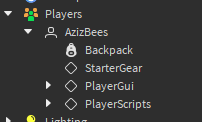# My Timer disappears whenever the player die

1. What do you want to achieve? Keep it simple and clear!
i want the timer to keep counting even if the player dies

2. What is the issue? Include screenshots / videos if possible!

3. What solutions have you tried so far? Did you look for solutions on the Developer Hub?
tried to disable "reset on spawn’ option from the gui but it didn’t work (the Timer didn’t even start counting when i touched the part)

this is the local script inside my Timer

``````local par=script.Parent

local timer=par:WaitForChild'Timer'
local timerlabel=timer:WaitForChild'Time'
local timertitle=timer:WaitForChild'Title'

local SetTime = tick()

local RunService = game:GetService("RunService")
-- edit this like 0 digit = 1, 1 digit = 1.0, 2 digit = 1.00 3 digit = 1.000 etc
local RationalDigits = 2

function connectplrtouch(pt,func)
pt.Touched:Connect(function(t)
local c=game.Players.LocalPlayer.Character
if c and t:IsDescendantOf(c) then
func()
end
end)
end

local timer_active=false
local timer_time=0

local prev
for _,d in pairs(workspace:GetDescendants()) do
if d.Name=='Timer_Start' then
local name=(d:FindFirstChild'Title' and d.Title:IsA'StringValue' and d.Title.Value) or ''
connectplrtouch(d,function()
par.Enabled=true
if prev~=d then
SetTime = tick()
prev=d
end
timertitle.Text=name
timerlabel.TextColor3=Color3.new(1, 1, 1)
SetTime = tick()
timer_active=true
end)
end
if d.Name=='Timer_Pause' then
connectplrtouch(d,function()
timerlabel.TextColor3=Color3.new(0, 1, 0)
if timer_active == true then
game.ReplicatedStorage.ApplyTime:FireServer(timerlabel.Text)
end
timer_active=false
end)
end
if d.Name=='Timer_Stop' then
connectplrtouch(d,function()
par.Enabled=false
timerlabel.TextColor3=Color3.new(0, 1, 0)
timer_active=false
SetTime = tick()
end)
end
end

local Accuracy = 10^RationalDigits
function TimerFunc()
if timer_active then

local Div1 = math.abs(tick() - SetTime)
local CalculatedIncrement = math.round(Div1*Accuracy)/Accuracy
for t=1,RationalDigits do
local i = t-1
local integer,predecimal = math.modf(CalculatedIncrement*(10^i))
local decimal = predecimal/(10^i)
if decimal == 0 then
end

end
local NewText = tostring(CalculatedIncrement)
NewText = NewText..v
end
timerlabel.Text=NewText

end
end
while true do
wait(.025)
TimerFunc()
end
``````
3 Likes

When a player dies, the gui, localscript, and any handles are destroyed.
You need to add a way to control this behavior.

For example, in your server code, the handle to the player is now nil. This is because the player died and the instance was deleted.

1 Like

Make sure to disable the property where the GUI resets on death. It will locate inside the screenGUI

1 Like

Turn off ResetOnSpawn on the ScreenGui

1 Like

bro i already said that i tried that:
** 1. What solutions have you tried so far? Did you look for solutions on the Developer Hub?
tried to disable "reset on spawn’ option from the gui but it didn’t work (the Timer didn’t even start counting when i touched the part)**

bro i already said that i tried that:

1. What solutions have you tried so far? Did you look for solutions on the Developer Hub?
tried to disable "reset on spawn’ option from the gui but it didn’t work (the Timer didn’t even start counting when i touched the part)

Weird, that should work. I’m thinking it’s going to be some kind of problem @RaterixRGL mentioned above. Investigate to see if the ScreenGui that controls the timer disappear from the player’s PlayerGui. It shouldn’t disappear if the gui is in StarterGui and ResetOnSpawn is off.

You should create value in local playerthis is the only script on the whole server since i made a new server just to test it,that’s why it’s weird to me

and how can i do that
can’t actually understant what you mean

Are the time values stored in the character? If they are stored, they will also be reset along with the counter

You can just find where you are disabling the ui and put prints there and then figure out how the code is being run and patch it.

i checked and it didn’t disappear when i turned “reset on spawn” off
as it didn’t work as well

ahhh i don’t know how to check that, it’s just a local script in a gui in StarterGui, and it appears on the PlayerGui

If ResetOnSpawn doesn’t work for you then try this.

``````local par = game.Players.LocalPlayer.PlayerGui:WaitForChild("TimerGui")
local timer=par:WaitForChild'Timer'
local timerlabel=timer:WaitForChild'Time'
local timertitle=timer:WaitForChild'Title'
local name
local timer_active=false

par = game.Players.LocalPlayer.PlayerGui:WaitForChild("TimerGui")
timer=par:WaitForChild'Timer'
timerlabel=timer:WaitForChild'Time'
timertitle=timer:WaitForChild'Title'

if timer_active then
timertitle.Text=name
par.Enabled=true
timerlabel.TextColor3=Color3.new(1, 1, 1)
end
end)

local SetTime = tick()

local RunService = game:GetService("RunService")
-- edit this like 0 digit = 1, 1 digit = 1.0, 2 digit = 1.00 3 digit = 1.000 etc
local RationalDigits = 2

function connectplrtouch(pt,func)
pt.Touched:Connect(function(t)
local c=game.Players.LocalPlayer.Character
if c and t:IsDescendantOf(c) then
func()
end
end)
end

local timer_time=0

local prev
for _,d in pairs(workspace:GetDescendants()) do
if d.Name=='Timer_Start' then
name=(d:FindFirstChild'Title' and d.Title:IsA'StringValue' and d.Title.Value) or ''
connectplrtouch(d,function()
par.Enabled=true
if prev~=d then
SetTime = tick()
prev=d
end
timertitle.Text=name
timerlabel.TextColor3=Color3.new(1, 1, 1)
SetTime = tick()
timer_active=true
end)
end
if d.Name=='Timer_Pause' then
connectplrtouch(d,function()
timerlabel.TextColor3=Color3.new(0, 1, 0)
if timer_active == true then
game.ReplicatedStorage.ApplyTime:FireServer(timerlabel.Text)
end
timer_active=false
end)
end
if d.Name=='Timer_Stop' then
connectplrtouch(d,function()
par.Enabled=false
timerlabel.TextColor3=Color3.new(0, 1, 0)
timer_active=false
SetTime = tick()
end)
end
end

local Accuracy = 10^RationalDigits
function TimerFunc()
if timer_active then

local Div1 = math.abs(tick() - SetTime)
local CalculatedIncrement = math.round(Div1*Accuracy)/Accuracy
for t=1,RationalDigits do
local i = t-1
local integer,predecimal = math.modf(CalculatedIncrement*(10^i))
local decimal = predecimal/(10^i)
if decimal == 0 then
end

end
local NewText = tostring(CalculatedIncrement)
NewText = NewText..v
end
timerlabel.Text=NewText

end
end
while true do
wait(.025)
TimerFunc()
end
``````

You have to place it into StarterPlayerScripts to dont make it reset.

you just fixed 2 issues for me with one solution